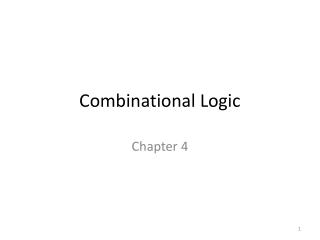DownloadDownload PresentationCombinational Logic

# Combinational Logic

Download Presentation## Combinational Logic

- - - - - - - - - - - - - - - - - - - - - - - - - - - E N D - - - - - - - - - - - - - - - - - - - - - - - - - - -
##### Presentation Transcript

1. Combinational Logic Chapter 4

2. Combinational Circuits

3. Combinational Circuits • Adders • Subtractors • Comparators • Decoders • Encoders • Multiplexers Available as MSI Circuits and as Standard Cells in VLSI (Bonus Assignment: Get one example of each type of combinational circuits in the CMOS family, 5 points for the second exam)

4. Analysis Procedure • Given a logical diagram, determine one or more of the following: • Boolean functions; • Truth table; • Explanation of circuit operation • Make sure the circuit is combinational, not sequential (No feedback loops)

5. Analysis Procedure • Label all gate outputs that are a function of input variables. Determine the Boolean function for each gate output • Label the gates that are a function of input variables and previously labeled gates. Find the Boolean functions for these gates • Repeat step 2 until output of circuits are obtained • By repeated substitution of previously defined functions, obtain the output Boolean functions in terms of input variables

6. Analysis Procedure

7. Analysis Procedure Step 1 Step 2 Steps 3 and 4

8. Analysis Procedure • Determine the number of input variables in the circuit. For inputs, form the possible input combinations and list the binary numbers from to in a table • Label the outputs of selected gates with arbitrary symbols • Obtain the truth table for the outputs of those gates which are a function of the input variables only • Proceed to obtain the truth table for the outputs of those gates which are a function of previously defined values until the columns for all outputs are determined

9. Analysis Procedure

10. Design Procedure • From the specifications of the circuit, determine the required number of inputs and outputs and assign a symbol to each • Derive the truth table that defines the required relationship between inputs and outputs • Obtain the simplified Boolean functions for each output as a function of the input variables • Draw the logical diagram and verify correctness of the design

11. Code conversion example

12. Code conversion example

13. Code conversion example

14. Code conversion example

15. Binary Adder-Subtractor Half adder

16. Half adder

17. Full adder

18. Full adder Implementation of full adder in sum-of-products

19. Full Adder

20. Full adder Implementation of full adder using two half adders and one or gate

21. Binary adder

22. Carry propagation Carry Generate Full Adder with and shown

23. Carry propagation Carry lookahead generator

24. Four-bit adder with carry lookahead Carry lookahead generator

25. Four-bit adder with carry lookahead

26. Binary subtractor

27. Overflow • Occurs only when adding two positive numbers or two negative numbers; • Overflow produces change in result sign Example: eight-bit adder Carry bits

28. Decimal Adder • Consider adding two decimal digits in BCD • Output sum cannot exceed 9+9+1=19 (the last 1 is the carry from previous digit)

29. Decimal Adder Carry Need correction Condition for correcting result

30. Decimal Adder

31. Binary Multiplier Exercise: Multiply Explain how you carried the multiplication out. How many bits at the output?

32. Binary Multiplier Two-bit multiplier

33. Binary Multiplier Exercise: With your neighbor classmate discuss its operation.

34. Magnitude Comparator Exercise: Discuss with your neighbor classmate and write down how you would compare two four-bit binary numbers and , where and . You should have three outputs corresponding to , , and . Explain how you determined each condition.

35. Magnitude Comparator Does this circuit correspond to what you wrote down in the exercise? Discuss again with your neighbor classmate the comparison of your result with this circuit.

36. Decoders Exercise: Minimize the functions for two of the eight output lines.

37. Decoders Exercise: Compare your function with the circuit. Three-to-eight-line decoder

38. Decoders decoder constructed with two decoders Exercise: Explain how this decoder works.

39. Combinational logic implementation Exercise: For the maps of the full adder shown above, express the sum (left) and the carry bit (right) as a sum of minterms.

40. Combinational Logic Implementation Compare in terms of propagation time and number of gates this Full Adder with the previously studied implementation.

41. Encoders

42. Priority encoder Highest priority Valid output

43. Priority encoder Exercise: obtain the function for

44. Priority encoder

45. Multiplexers • Selects binary information from one of many input lines • Directs input line to output, controlled by a set of selection lines • input lines and selection lines

46. Multiplexers Two-to-one-line multiplexer

47. Multiplexers Four-to-one-line multiplexer

48. Multiplexers Quadruple two-to-one-line multiplexer

49. Boolean function implementation • Multiplexer is essentially a decoder with OR gates • Thus can implement Boolean functions, similar to decoders • Minterms generated by circuit associated with selection inputs • Individual minterms can be selected by data inputs • Boolean function of variables and data inputs